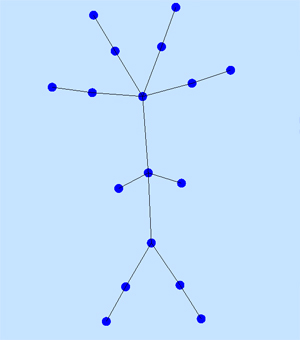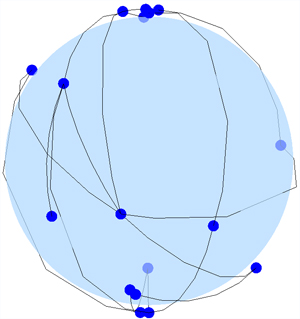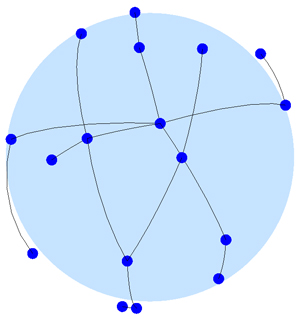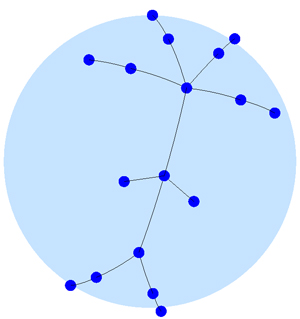# Non-Euclidean Spring Embedders

### Luksch Christian, 0525392 Tanzer Andrea, 0325618 SS 2007, Informationsvisualisierung

Based on the paper Non-Euclidean Spring Embedders by Stephen G. Kobourov and Kevin WamplerScreenshot of graphs in spherical space.

Spring Embedders is a programm written in C#. It is a program for visualising graphs. You can either load existing Nodes (node + graphlayout or just nodes) into the program, or create your own graph.

### Motivation

• Automatically calculate a well structured graph layout in spherical, hyperbolical or euclidean geometry.
• Better visualisation of large classes of graphs.
• Efficient spatial usage

• ### Spring Embedders

"Some of the most flexible algorithms for calculating layouts of simple undirected graphs belong to a class known as forcedirected algorithms. Also known as spring embedders, such algorithms calculate the layout of a graph using only information contained within the structure of the graph itself." 
• Calculates the graph with 2 forces: Attraction Force and Repulsion Force
• Minimize forces affecting graph

• #### Attraction Force 

d² / k

d... Distance between nodes
k... Optimal distance between nodes (calculated automatically from available surface and number of nodes)

#### Repulsion Force 

k²/ d

d... Distance between nodes
k... Optimal distance between nodes (calculated automatically from available surface and number of nodes)

#### Features

• graphs in spherical, hyperbolical and euclidean geometry
• zooming, panning and rotation on layouts possible
• dragging nodes on layout in view mode
• intuitive user interface design
• save graphs (layout + nodes or just nodes)
• open saved graphs
• view and edit mode
• graph information (number of nodes, number of edges, average tension that takes effect on the graph, ..)
• realtime simulation update OR step by step simulation update
• chance visual parameters (node color, edge color, layout color)
• name nodes, draw captions
• GPU acceleration

• #### File Format

You can either save graph layouts or nodes and load existing graphs. Now i want to introduce you to our file format- it is really simple! I saved a graph with its layout and without. I will explain it to you using this graph: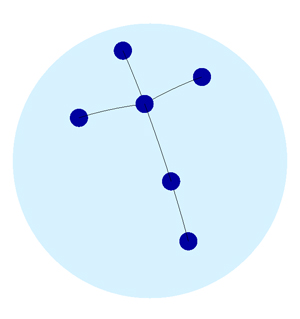Graph only:

; Graph file
;###########
;
;Nodes:
NODE: ID=1; CAPTION="";
NODE: ID=2; CAPTION="";
NODE: ID=3; CAPTION="";
NODE: ID=4; CAPTION="";
NODE: ID=5; CAPTION="";
NODE: ID=6; CAPTION="";
;Edges:
EDGE: START=1; END=2;
EDGE: START=2; END=3;
EDGE: START=2; END=4;
EDGE: START=2; END=6;
EDGE: START=5; END=6;

Each node has its own node-id. I did not name any node so the caption is just "" (empty). So, if you want to load this file, our algorithm just reads every line. On the one hand NODE means a node with its saved id shall be drawn, on the other hand EDGE means a edge from START (node id) to END (other node id) shall be drawn. When all this is done our algorithm calculates a well structured graph in whatever layout you are in.

Graph + Layout:

; Graph file
;###########
;
;General:
LAYOUT: Spheric
;Nodes:
NODE: ID=1; CAPTION=""; X=3,343782; Y=2,332447;
NODE: ID=2; CAPTION=""; X=2,688113; Y=2,29821;
NODE: ID=3; CAPTION=""; X=2,921685; Y=1,878323;
NODE: ID=4; CAPTION=""; X=2,596091; Y=2,759698;
NODE: ID=5; CAPTION=""; X=1,711079; Y=1,739977;
NODE: ID=6; CAPTION=""; X=2,081431; Y=2,03813;
;Edges:
EDGE: START=1; END=2;
EDGE: START=2; END=3;
EDGE: START=2; END=4;
EDGE: START=2; END=6;
EDGE: START=5; END=6;

First the layout is specified. In our case we saved our graph in spherical layout. So our algorithm switches the layout to spherical when loading the file (if it isn't already spherical layout) Then nodes are loaded. This time we also have to read x and y positions in, because we saved the graph with the exact position so we can load it exact the same way we saved it. Finally, we just have to calculate a well structured graph if it isn't already calculated (i.e.: saved during minimizing forces).

### Sample graph

• First, draw some nodes and connect them somehow (edit mode)
• Then, change to spherical or hyperbolical layout (you can see how a well structured graph is calculated)
• Last, you can save your created graph.

•